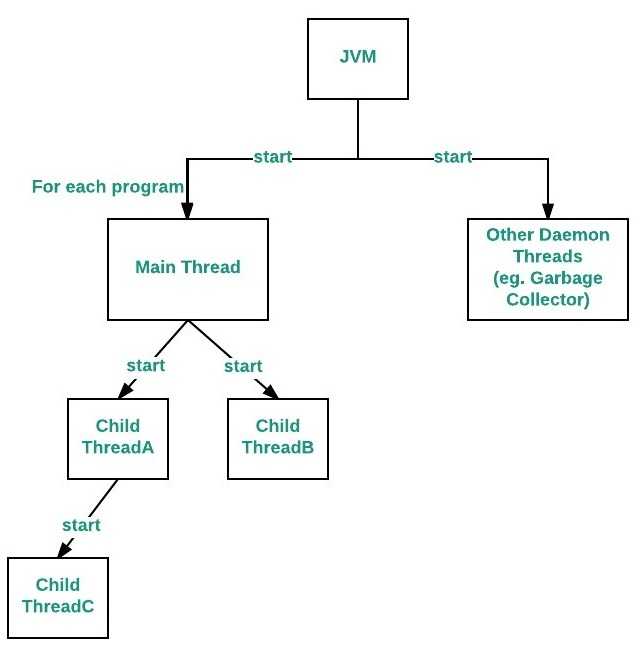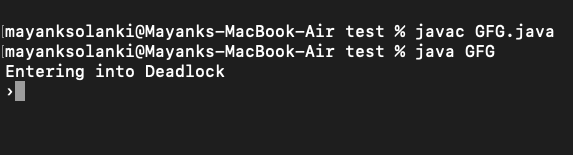Open in App
Not now

• Difficulty Level : Easy
• Last Updated : 21 Sep, 2021

Java provides built-in support for multithreaded programming. A multi-threaded program contains two or more parts that can run concurrently. Each part of such a program is called a thread, and each thread defines a separate path of execution.
When a Java program starts up, one thread begins running immediately. This is usually called the main thread of our program because it is the one that is executed when our program begins.

There are certain properties associated with the main thread which are as follows:

• It is the thread from which other “child” threads will be spawned.
• Often, it must be the last thread to finish execution because it performs various shutdown actions

The flow diagram is as follows:The main thread is created automatically when our program is started. To control it we must obtain a reference to it. This can be done by calling the method currentThread( ) which is present in Thread class. This method returns a reference to the thread on which it is called. The default priority of Main thread is 5 and for all remaining user threads priority will be inherited from parent to child.

Example

## Java

 `// Java program to control the Main Thread`   `// Importing required classes` `import` `java.io.*;` `import` `java.util.*;`   `// Class 1` `// Main class extending thread class` `public` `class` `Test ``extends` `Thread {`   `    ``// Main driver method` `    ``public` `static` `void` `main(String[] args)` `    ``{`   `        ``// Getting reference to Main thread` `        ``Thread t = Thread.currentThread();`   `        ``// Getting name of Main thread` `        ``System.out.println(``"Current thread: "` `                           ``+ t.getName());`   `        ``// Changing the name of Main thread` `        ``t.setName(``"Geeks"``);` `        ``System.out.println(``"After name change: "` `                           ``+ t.getName());`   `        ``// Getting priority of Main thread` `        ``System.out.println(``"Main thread priority: "` `                           ``+ t.getPriority());`   `        ``// Setting priority of Main thread to MAX(10)` `        ``t.setPriority(MAX_PRIORITY);`   `        ``// Print and display the main thread priority` `        ``System.out.println(``"Main thread new priority: "` `                           ``+ t.getPriority());`   `        ``for` `(``int` `i = ``0``; i < ``5``; i++) {` `            ``System.out.println(``"Main thread"``);` `        ``}`   `        ``// Main thread creating a child thread` `        ``Thread ct = ``new` `Thread() {` `            ``// run() method of a thread` `            ``public` `void` `run()` `            ``{`   `                ``for` `(``int` `i = ``0``; i < ``5``; i++) {` `                    ``System.out.println(``"Child thread"``);` `                ``}` `            ``}` `        ``};`   `        ``// Getting priority of child thread` `        ``// which will be inherited from Main thread` `        ``// as it is created by Main thread` `        ``System.out.println(``"Child thread priority: "` `                           ``+ ct.getPriority());`   `        ``// Setting priority of Main thread to MIN(1)` `        ``ct.setPriority(MIN_PRIORITY);`   `        ``System.out.println(``"Child thread new priority: "` `                           ``+ ct.getPriority());`   `        ``// Starting child thread` `        ``ct.start();` `    ``}` `}`   `// Class 2` `// Helper class extending Thread class` `// Child Thread class` `class` `ChildThread ``extends` `Thread {`   `    ``@Override` `public` `void` `run()` `    ``{`   `        ``for` `(``int` `i = ``0``; i < ``5``; i++) {`   `            ``// Print statement whenever child thread is` `            ``// called` `            ``System.out.println(``"Child thread"``);` `        ``}` `    ``}` `}`

Output

```Current thread: main
After name change: Geeks

Now let us discuss the relationship between the main() method and the main thread in Java. For each program, a Main thread is created by JVM(Java Virtual Machine). The “Main” thread first verifies the existence of the main() method, and then it initializes the class. Note that from JDK 6, main() method is mandatory in a standalone java application.

We can create a deadlock by just using the Main thread, i.e. by just using a single thread.

Example

## Java

 `// Java program to demonstrate deadlock` `// using Main thread`   `// Main class` `public` `class` `GFG {`   `  ``// Main driver method` `  ``public` `static` `void` `main(String[] args) {`   `    ``// Try block to check for exceptions` `    ``try` `{`   `      ``// Print statement` `      ``System.out.println(``"Entering into Deadlock"``);`   `      ``// Joining the current thread` `      ``Thread.currentThread().join();`   `      ``// This statement will never execute` `      ``System.out.println(``"This statement will never execute"``);` `    ``}`   `    ``// Catch block to handle the exceptions` `    ``catch` `(InterruptedException e) {`   `      ``// Display the exception along with line number` `      ``// using printStackTrace() method` `      ``e.printStackTrace();` `    ``}` `  ``}` `}`

Output:Output explanation: